Browse by Topic

# How Optimistic Do You Want to Be? Bayesian Neural Network Regression with Prediction Errors

Neural networks are very well known for their uses in machine learning, but can be used as well in other, more specialized topics, like regression. Many people would probably first associate regression with statistics, but let me show you the ways in which neural networks can be helpful in this field. They are especially useful if the data you’re interested in doesn’t follow an obvious underlying trend you can exploit, like in polynomial regression.

In a sense, you can view neural network regression as a kind of intermediary solution between true regression (where you have a fixed probabilistic model with some underlying parameters you need to find) and interpolation (where your goal is mostly to draw an eye-pleasing line between your data points). Neural networks can get you something from both worlds: the flexibility of interpolation and the ability to produce predictions with error bars like when you do regression.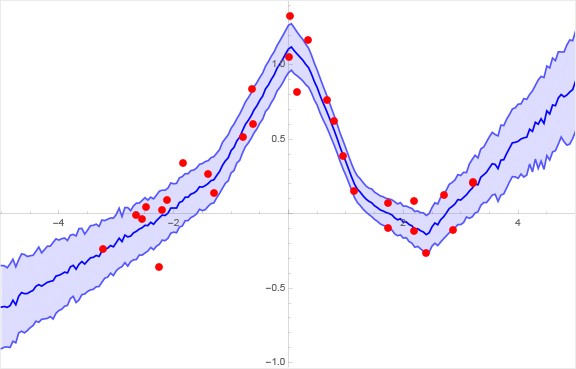For those of you who already know about neural networks, I can give a very brief hint as to how this works: you build a randomized neural network with dropout layers that you train like you normally would, but after training you don’t deactivate the dropout layers and keep using them to sample the network several times while making predictions to get a measure of the errors. Don’t worry if that sentence didn’t make sense to you yet, because I will explain all of this in more detail.

## Ordinary Neural Network Regression

To start, let’s do some basic neural network regression on the following data I made by taking points on a bell curve (e.g. the function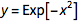) and adding random numbers to it: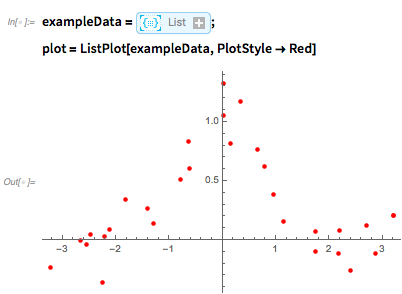✕ ```exampleData = {{-1.8290606952826973`, 0.34220332868351117`}, {-0.6221091101205225`, 0.6029615713235724`}, {-1.2928624443456638`, 0.14264805848673934`}, {1.7383127604822395`, \ -0.09676233458358859`}, {2.701795903782372`, 0.1256597483577385`}, {1.7400006797156493`, 0.07503425036465608`}, {-0.6367237544480613`, 0.8371547667282598`}, {-2.482802633037993`, 0.04691691595492773`}, {0.9566109777301293`, 0.3860569423794188`}, {-2.551790012296368`, \ -0.037340684890464014`}, {0.6626176509888584`, 0.7670620756823968`}, {2.865357628008809`, -0.1120949485036743`}, \ {0.024445094773154707`, 1.3288343886644758`}, {-2.6538667331049197`, \ -0.005468132072381475`}, {1.1353110951218213`, 0.15366247144719652`}, {3.209853579579198`, 0.20621896435600656`}, {0.13992534568622972`, 0.8204487134187859`}, {2.4013110392840886`, \ -0.26232722849881523`}, {-2.1199290467312526`, 0.09261482926621102`}, {-2.210336371360782`, 0.02664895740254644`}, {0.33732886898809156`, 1.1701573388517288`}, {-2.2548343241910374`, \ -0.3576908508717164`}, {-1.4077788877461703`, 0.269393680956761`}, {3.210242875591371`, 0.21099679051999695`}, {0.7898064016052615`, 0.6198835029596128`}, {2.1835077887328893`, 0.08410415228550497`}, {0.008631687647122632`, 1.0501425654209409`}, {2.1792531502694334`, \ -0.11606480328877161`}, {-3.231947584552822`, -0.2359904673791076`}, \ {-0.7980615888830211`, 0.5151437742866803`}} plot = ListPlot[exampleData, PlotStyle -> Red]```

A regression neural network is basically a chain of alternating linear and nonlinear layers: the linear layers give your net a lot of free parameters to work with, while the nonlinear layers make sure that things don’t get boring. Common examples of nonlinear layers are the hyperbolic tangent, logistic sigmoid and the ramp function. For simplicity, I will stick with the Ramp nonlinearity, which simply puts kinks into straight lines (meaning that you get regressions that are piecewise linear):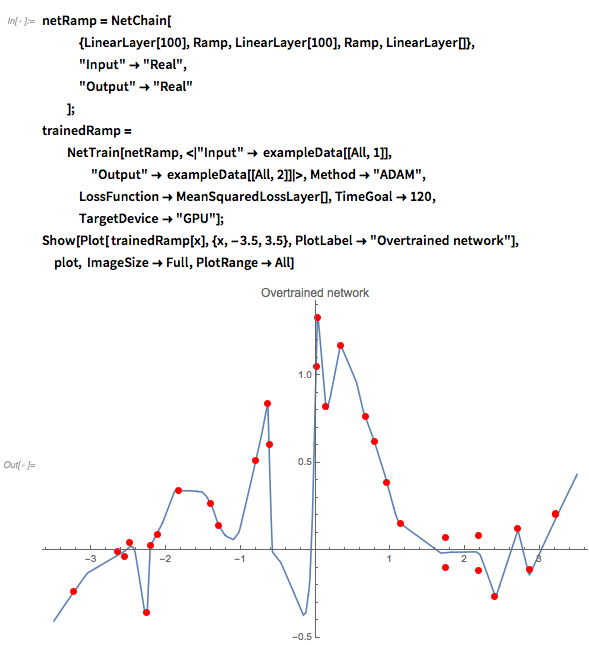✕ ```netRamp = NetChain[ {LinearLayer, Ramp, LinearLayer, Ramp, LinearLayer[]}, "Input" -> "Real", "Output" -> "Real" ]; trainedRamp = NetTrain[netRamp, <|"Input" -> exampleData[[All, 1]], "Output" -> exampleData[[All, 2]]|>, Method -> "ADAM", LossFunction -> MeanSquaredLossLayer[], TimeGoal -> 120, TargetDevice -> "GPU"]; Show[Plot[ trainedRamp[x], {x, -3.5, 3.5}, PlotLabel -> "Overtrained network"], plot, ImageSize -> Full, PlotRange -> All]```

As you can see, the network more or less just follows the points because it doesn’t understand the difference between the trend and the noise in the data. In the range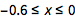above, the mix-up between trend and noise is particularly bad. The longer you train the network and the larger your linear layer, the stronger this effect will be. Obviously this is not what you want, since you’re really interested in fitting the trend of the data. Besides: if you really want to fit noise, you could just use interpolation instead. To prevent this overfitting of the data, you regularize (as explained in this tutorial) the network by using any or all of the following: a ValidationSet,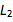regularization or a DropoutLayer. I will focus on theregularization coefficient and on dropout layers (in the next section you’ll see why), so let me briefly explain how they work:

•regularization is also commonly known in neural networks as weight decay. It’s a method that penalizes the network for using large weights, which prevents it from over-complicating the regression curve. The size of this penalty is scaled by a parameter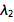called the regularization coefficient: the larger this coefficient, the simpler the network is forced to be.
• Dropout, on the other hand, randomizes the network during training to make sure that it can’t just fit the noise in the data. A network with dropout layers is also called a stochastic network (though dropout is not the only way people can make a network stochastic). The amount of randomness is controlled by the dropout probability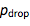(which is commonly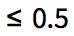).

To get a feeling of how these two methods regularize the regression, I made the following parameter sweeps of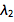and: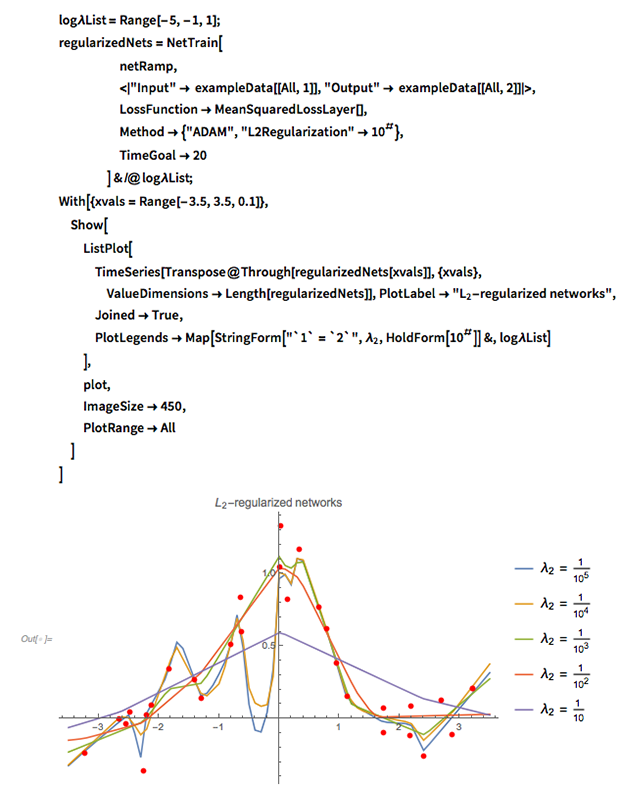✕ ```log\[Lambda]List = Range[-5, -1, 1]; regularizedNets = NetTrain[ netRamp, <|"Input" -> exampleData[[All, 1]], "Output" -> exampleData[[All, 2]]|>, LossFunction -> MeanSquaredLossLayer[], Method -> {"ADAM", "L2Regularization" -> 10^#}, TimeGoal -> 20 ] & /@ log\[Lambda]List; With[{xvals = Range[-3.5, 3.5, 0.1]}, Show[ ListPlot[ TimeSeries[Transpose@Through[regularizedNets[xvals]], {xvals}, ValueDimensions -> Length[regularizedNets]], PlotLabel -> "\!\(\*SubscriptBox[\(L\), \(2\)]\)-regularized networks", Joined -> True, PlotLegends -> Map[StringForm["`1` = `2`", Subscript[\[Lambda], 2], HoldForm[10^#]] &, log\[Lambda]List] ], plot, ImageSize -> 450, PlotRange -> All ] ]```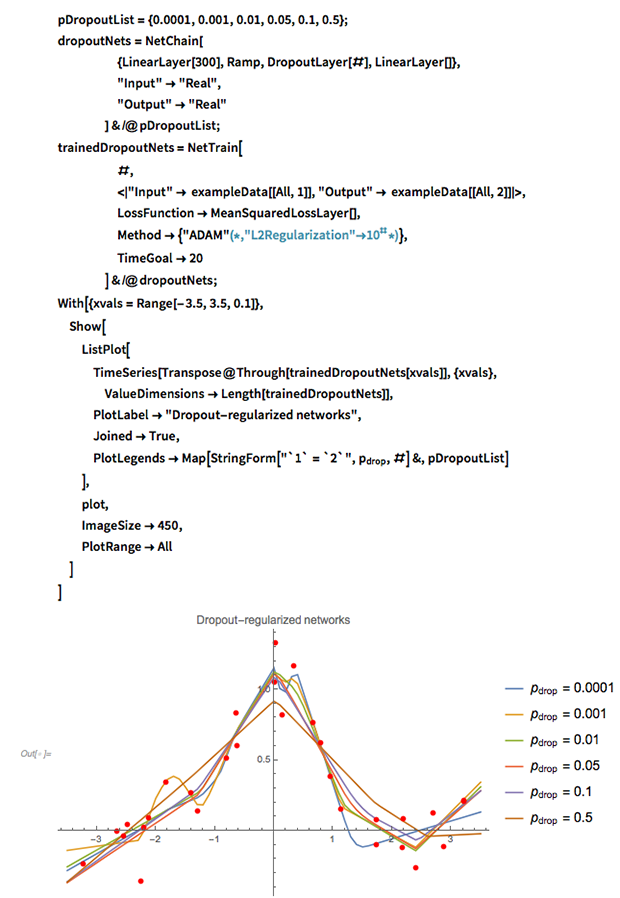✕ ```pDropoutList = {0.0001, 0.001, 0.01, 0.05, 0.1, 0.5}; dropoutNets = NetChain[ {LinearLayer, Ramp, DropoutLayer[#], LinearLayer[]}, "Input" -> "Real", "Output" -> "Real" ] & /@ pDropoutList; trainedDropoutNets = NetTrain[ #, <|"Input" -> exampleData[[All, 1]], "Output" -> exampleData[[All, 2]]|>, LossFunction -> MeanSquaredLossLayer[], Method -> {"ADAM"(*,"L2Regularization"\[Rule]10^#*)}, TimeGoal -> 20 ] & /@ dropoutNets; With[{xvals = Range[-3.5, 3.5, 0.1]}, Show[ ListPlot[ TimeSeries[Transpose@Through[trainedDropoutNets[xvals]], {xvals}, ValueDimensions -> Length[trainedDropoutNets]], PlotLabel -> "Dropout-regularized networks", Joined -> True, PlotLegends -> Map[StringForm["`1` = `2`", Subscript[p, drop], #] &, pDropoutList] ], plot, ImageSize -> 450, PlotRange -> All ] ]```

To summarize:

• Regular prediction neural networks (NNs) usually consist of an alternating linear layer and nonlinear layers.
• The network can be made more flexible by using linear layers of a higher dimension or by simply making the chain longer.
• Because your network is very flexible, you need to apply some form of regularization to make sure you’re not just fitting noise.

## Neural Network Regression with Error Bands

Both regularization methods mentioned previously were originally proposed as ad hoc solutions to the overfitting problem. However, recent work has shown that there are actually very good fundamental mathematical reasons why these methods work. Even more importantly, it has been shown that you can use them to do better than just produce a regression line! For those of you who are interested, I suggest reading this blog post by Yarin Gal. His thesis “Uncertainty in Deep Learning” is also well worth a look and is the main source for what follows in the rest of this post.

As it turns out, there is a link between stochastic regression neural networks and Gaussian processes, which are free-form regression methods that let you predict values and put error bands on those predictions. To do this, we need to consider neural network regression as a proper Bayesian inference procedure. Normally, Bayesian inference is quite computationally expensive, but as it conveniently turns out, you can do an approximate inference with minimal extra effort on top of what I already did above.

The basic idea is to use dropout layers to create a noisy neural network that is trained on the data as normal. However, I’m also going to use the dropout layers when doing predictions: for every value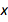where I need a prediction, I will sample the network multiple times to get a sense of the errors in the predictions.

Furthermore, it’s good to keep in mind that you, as a newly converted Bayesian, are also dealing with priors. In particular, the network weights are now random variables with a prior distribution and a posterior distribution (i.e. the distributions before and after learning). This may sound rather difficult, so let me try to answer two questions you may have at this point:

Q1: Does that mean that I actually have to think hard about my prior now?
A1: No, not really, because it simply turns out that our old friend, the regularization coefficient, is really just the inverse standard deviation of the network prior weights: if you choose a larger, that means you’re only allowing small network weights.
Q2: So what about the posterior distribution of the weights? Don’t I have to integrate the predictions over the posterior weight distribution to get a posterior predictive distribution?
A2: Yes, you do, and that’s exactly what you do (at least approximately) when you sample the trained network with the dropout layers active. The sampling of the network is just a form of Monte Carlo integration over the posterior distribution.

So as you can see, being a Bayesian here really just means giving things a different name without having to change your way of doing things very much.

## Regression with a Constant Noise Level

Let’s start with the simplest type of regression in which the noise level of the data is assumed constant across the x axis. This is also called homoscedastic regression (as opposed to heteroscedastic regression, where the noise is a function of x). It does not, however, mean that the prediction error will also be constant: the prediction error depends on the noise level but also on the uncertainty in the network weights.

So let’s get to it and see how this works out, shall we? First I will define my network with a dropout layer. Normally you’d put a dropout layer before every linear layer, but since the input is just a number, I’m omitting the first dropout layer: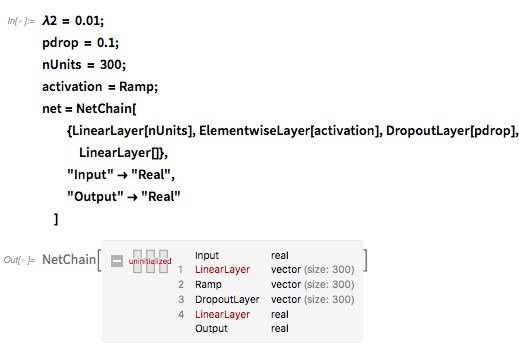✕ ```\[Lambda]2 = 0.01; pdrop = 0.1; nUnits = 300; activation = Ramp; net = NetChain[ {LinearLayer[nUnits], ElementwiseLayer[activation], DropoutLayer[pdrop], LinearLayer[]}, "Input" -> "Real", "Output" -> "Real" ]```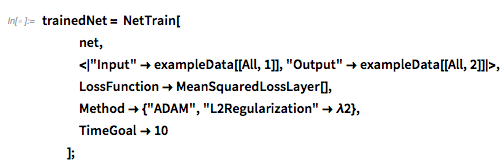✕ ```trainedNet = NetTrain[ net, <|"Input" -> exampleData[[All, 1]], "Output" -> exampleData[[All, 2]]|>, LossFunction -> MeanSquaredLossLayer[], Method -> {"ADAM", "L2Regularization" -> \[Lambda]2}, TimeGoal -> 10 ];```

Next, we need to produce predictions from this model. To calibrate the model, you need to provide a prior length scale l that expresses your belief in how correlated the data is over a distance (just like in Gaussian process regression). Together with theregularization coefficient, the dropout probability p and the number of training data points N, you have to add the following variance to the sample variance of the network: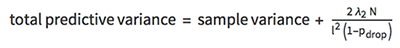The following function takes a trained net and samples it multiple times with the dropout layers active (using NetEvaluationMode → "Train"). It then constructs a time series object of the –1, 0 and +1σ bands of the predictions: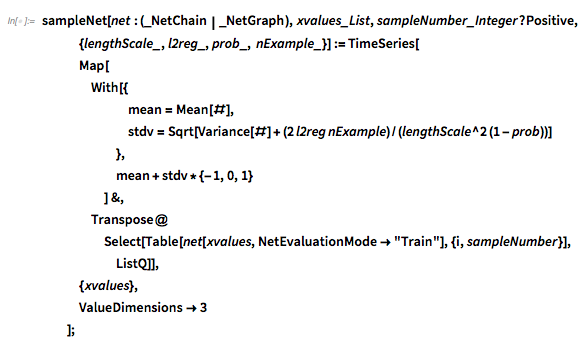✕ ```sampleNet[net : (_NetChain | _NetGraph), xvalues_List, sampleNumber_Integer?Positive, {lengthScale_, l2reg_, prob_, nExample_}] := TimeSeries[ Map[ With[{ mean = Mean[#], stdv = Sqrt[Variance[#] + (2 l2reg nExample)/(lengthScale^2 (1 - prob))] }, mean + stdv*{-1, 0, 1} ] &, Transpose@ Select[Table[ net[xvalues, NetEvaluationMode -> "Train"], {i, sampleNumber}], ListQ]], {xvalues}, ValueDimensions -> 3 ];```

Now we can go ahead and plot the predictions with 1σ error bands. The prior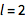seems to work reasonably well, though in real applications you’d need to calibrate it with a validation set (just like you would withand p).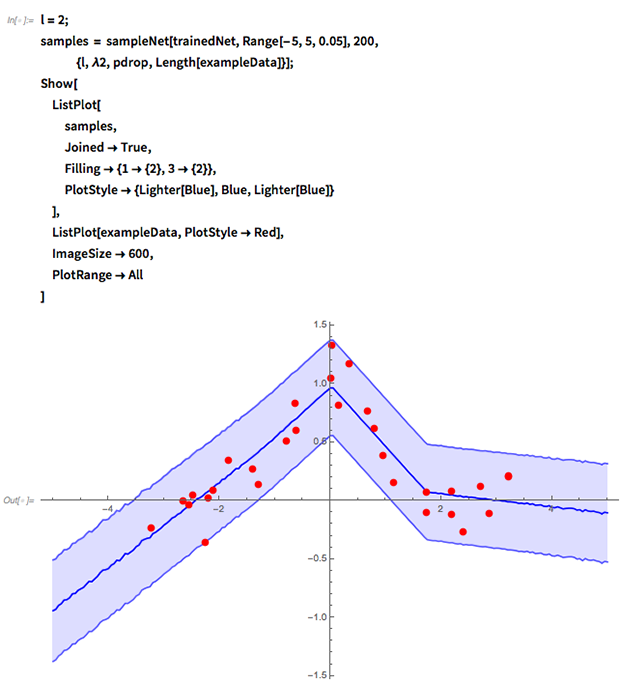✕ ```l = 2; samples = sampleNet[trainedNet, Range[-5, 5, 0.05], 200, {l, \[Lambda]2, pdrop, Length[exampleData]}]; Show[ ListPlot[ samples, Joined -> True, Filling -> {1 -> {2}, 3 -> {2}}, PlotStyle -> {Lighter[Blue], Blue, Lighter[Blue]} ], ListPlot[exampleData, PlotStyle -> Red], ImageSize -> 600, PlotRange -> All ]```

As you can see, the network has a tendency to do linear extrapolation due to my choice of the ramp nonlinearity. Picking different nonlinearities will lead to different extrapolation behaviors. In terms of Gaussian process regression, the choice of your network design influences the effective covariance kernel you’re using.

If you’re curious to see how the different network parameters influence the look of the regression, skip down a few paragraphs and try the manipulates, where you can interactively train your own network on data you can edit on the fly.

## Regression on Data with Varying Noise Levels

In heteroscedastic regression, you let the neural net try and find the noise level for itself. This means that the regression network outputs two numbers instead of one: a mean and a standard deviation. However, since the outputs of the network are real numbers, it’s easier if you use the log-precision instead of the standard deviation: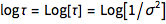: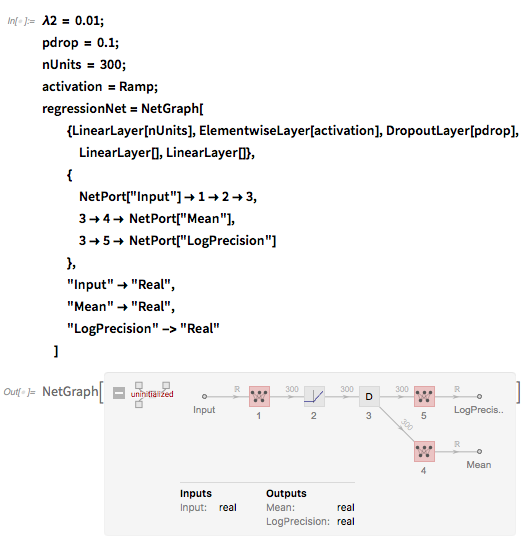✕ ```\[Lambda]2 = 0.01; pdrop = 0.1; nUnits = 300; activation = Ramp; regressionNet = NetGraph[ {LinearLayer[nUnits], ElementwiseLayer[activation], DropoutLayer[pdrop], LinearLayer[], LinearLayer[]}, { NetPort["Input"] -> 1 -> 2 -> 3, 3 -> 4 -> NetPort["Mean"], 3 -> 5 -> NetPort["LogPrecision"] }, "Input" -> "Real", "Mean" -> "Real", "LogPrecision" -> "Real" ]```

Next, instead of using a MeanSquaredLossLayer to train the network, you minimize the negative log-likelihood of the observed data. Again, you replace σ with the log of the precision and multiply everything by 2 to be in agreement with the convention of MeanSquaredLossLayer.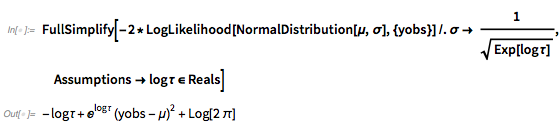✕ ```FullSimplify[-2* LogLikelihood[ NormalDistribution[\[Mu], \[Sigma]], {yobs}] /. \[Sigma] -> 1/ Sqrt[Exp[log\[Tau]]], Assumptions -> log\[Tau] \[Element] Reals]```

Discarding the constant term gives us the following loss: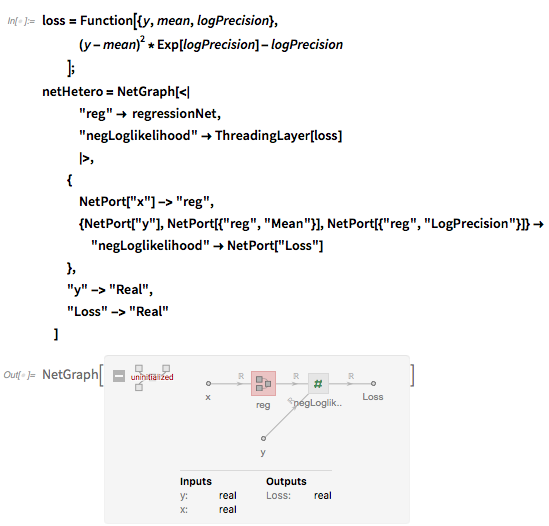✕ ```loss = Function[{y, mean, logPrecision}, (y - mean)^2*Exp[logPrecision] - logPrecision ]; netHetero = NetGraph[<| "reg" -> regressionNet, "negLoglikelihood" -> ThreadingLayer[loss] |>, { NetPort["x"] -> "reg", {NetPort["y"], NetPort[{"reg", "Mean"}], NetPort[{"reg", "LogPrecision"}]} -> "negLoglikelihood" -> NetPort["Loss"] }, "y" -> "Real", "Loss" -> "Real" ]```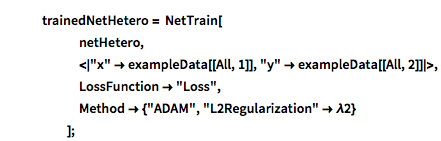✕ ```trainedNetHetero = NetTrain[ netHetero, <|"x" -> exampleData[[All, 1]], "y" -> exampleData[[All, 2]]|>, LossFunction -> "Loss", Method -> {"ADAM", "L2Regularization" -> \[Lambda]2} ];```

Again, the predictions are sampled multiple times. The predictive variance is now the sum of the variance of the predicted mean + mean of the predicted variance. The priors no longer influence the variance directly, but only through the network training: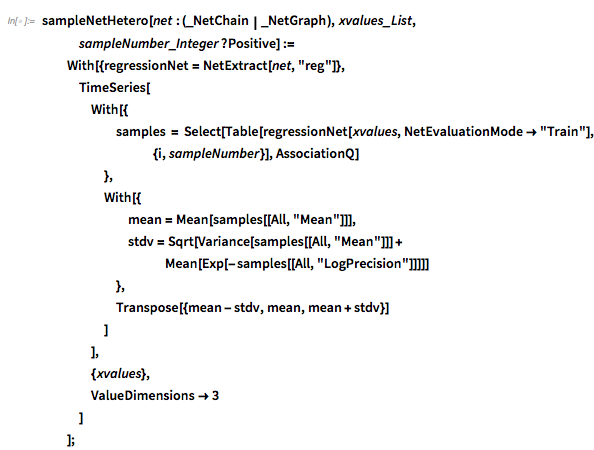✕ ```sampleNetHetero[net : (_NetChain | _NetGraph), xvalues_List, sampleNumber_Integer?Positive] := With[{regressionNet = NetExtract[net, "reg"]}, TimeSeries[ With[{ samples = Select[Table[ regressionNet[xvalues, NetEvaluationMode -> "Train"], {i, sampleNumber}], AssociationQ] }, With[{ mean = Mean[samples[[All, "Mean"]]], stdv = Sqrt[Variance[samples[[All, "Mean"]]] + Mean[Exp[-samples[[All, "LogPrecision"]]]]] }, Transpose[{mean - stdv, mean, mean + stdv}] ] ], {xvalues}, ValueDimensions -> 3 ] ];```

Now you can plot the predictions with 1σ error bands: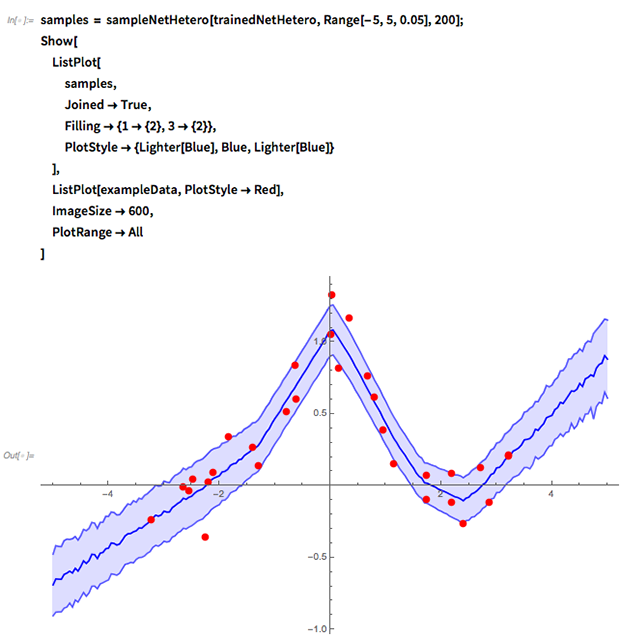✕ ```samples = sampleNetHetero[trainedNetHetero, Range[-5, 5, 0.05], 200]; Show[ ListPlot[ samples, Joined -> True, Filling -> {1 -> {2}, 3 -> {2}}, PlotStyle -> {Lighter[Blue], Blue, Lighter[Blue]} ], ListPlot[exampleData, PlotStyle -> Red], ImageSize -> 600, PlotRange -> All ]```

Of course, it’s still necessary to do validation of this network; one network architecture might be much better suited to the data at hand than another, so there is still the need to use validation sets to decide which model you have to use and with what parameters. Attached to the end of this blog post, you’ll find a notebook with an interactive demo of the regression method I just showed. With this code, you can find out for yourself how the different model parameters influence the predictions of the network.

## Optimistic or Pessimistic Error Bars? Introducing the Alpha-Divergence Loss Function

The code in this section shows how to implement the loss function described in the paper “Dropout Inference in Bayesian Neural Networks with Alpha-Divergences” by Li and Gal. For an interpretation of the α parameter used in this work, see e.g. figure 2 in “Black-Box α-Divergence Minimization” by Hernández-Lobato et al (2016).

In the paper by Li and Gal, the authors propose a modified loss function ℒ for a stochastic neural network to solve a weakness of the standard loss function I used above: it tends to underfit the posterior and give overly optimistic predictions. Optimistic predictions are a problem: when you fit your data to try and get a sense of what the real world might give you, you don’t want to be thrown a curveball afterwards.

During training, the training inputs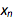(with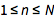indexing the training examples) are fed through the network K times to sample the outputs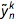and compared to the training outputs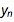. Given a particular standard loss function l (e.g. mean square error, negative log likelihood, cross-entropy) and regularization function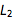for the weights θ, the modified loss function ℒ is given as: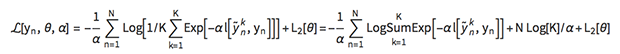The parameter α is the divergence parameter, which is typically tuned to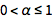(though you can pick other values as well, if you want). It can be thought of as a “pessimism” parameter: the higher it is, the more the network will tend to err on the side of caution and the larger error estimates. Practically speaking, a higher α parameter makes the loss function more lenient to the presence of large losses among the K samples, meaning that after training the network will produce a larger spread of predictions when sampled. Literature seems to suggest that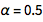is a pretty good value to start with. In the limit α→0, the LogSumExp simply becomes the sample average over K losses.

As can be seen, we need to sample the network several times during training. We can accomplish this with NetMapOperator. As a simple example, suppose we want to apply a dropout layer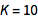times to the same input. To do this, we duplicate the input and then wrap a NetMapOperator around the dropout layer and map it over the duplicated input: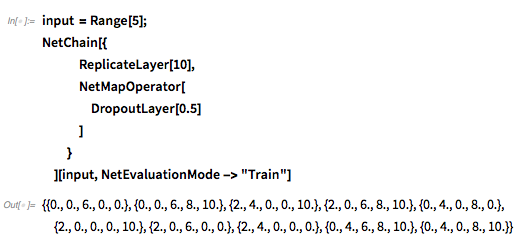✕ ```input = Range; NetChain[{ ReplicateLayer, NetMapOperator[ DropoutLayer[0.5] ] } ][input, NetEvaluationMode -> "Train"]```

Next, define a net that will try to fit the data points with a normal distribution like in the previous heteroscedastic example. The output of the net is now a length-2 vector with the mean and the log precision (we can’t have two output ports because we’re going to have wrap the whole thing into NetMapOperator):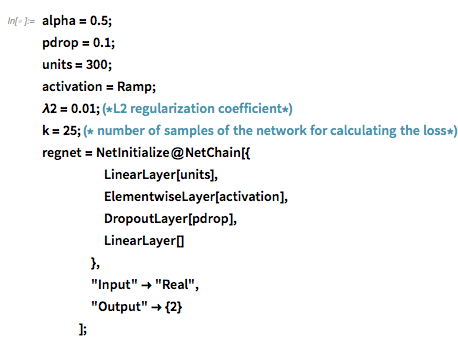✕ ```alpha = 0.5; pdrop = 0.1; units = 300; activation = Ramp; \[Lambda]2 = 0.01; (*L2 regularization coefficient*) k = 25; (* number of samples of the network for calculating the loss*) regnet = NetInitialize@NetChain[{ LinearLayer[units], ElementwiseLayer[activation], DropoutLayer[pdrop], LinearLayer[] }, "Input" -> "Real", "Output" -> {2} ];```

You will also need a network element to calculate the LogSumExp operator that aggregates the losses of the different samples of the regression network. I implemented the α-weighted LogSumExp by factoring out the largest term before feeding the vector into the exponent to make it more numerically stable. Note that I’m ignoring the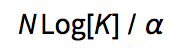term since it’s a constant for the purpose of training the network.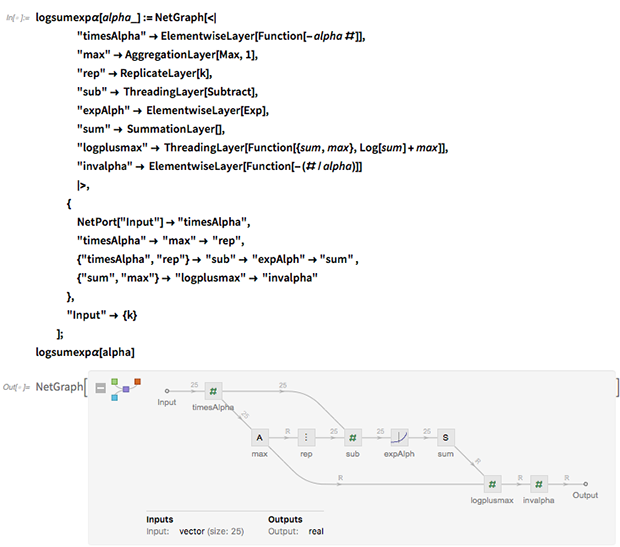✕ ```logsumexp\[Alpha][alpha_] := NetGraph[<| "timesAlpha" -> ElementwiseLayer[Function[-alpha #]], "max" -> AggregationLayer[Max, 1], "rep" -> ReplicateLayer[k], "sub" -> ThreadingLayer[Subtract], "expAlph" -> ElementwiseLayer[Exp], "sum" -> SummationLayer[], "logplusmax" -> ThreadingLayer[Function[{sum, max}, Log[sum] + max]], "invalpha" -> ElementwiseLayer[Function[-(#/alpha)]] |>, { NetPort["Input"] -> "timesAlpha", "timesAlpha" -> "max" -> "rep", {"timesAlpha", "rep"} -> "sub" -> "expAlph" -> "sum" , {"sum", "max"} -> "logplusmax" -> "invalpha" }, "Input" -> {k} ]; logsumexp\[Alpha][alpha]```

Define the network that will be used for training: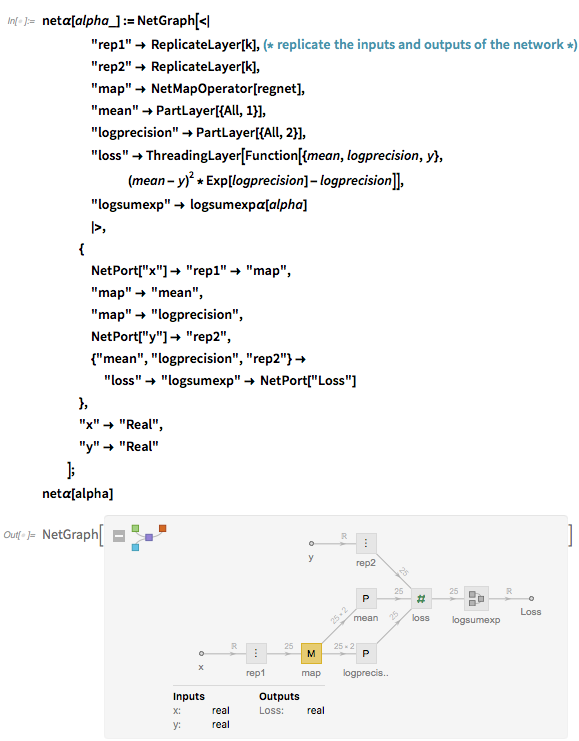✕ ```net\[Alpha][alpha_] := NetGraph[<| "rep1" -> ReplicateLayer[k],(* replicate the inputs and outputs of the network *) "rep2" -> ReplicateLayer[k], "map" -> NetMapOperator[regnet], "mean" -> PartLayer[{All, 1}], "logprecision" -> PartLayer[{All, 2}], "loss" -> ThreadingLayer[ Function[{mean, logprecision, y}, (mean - y)^2*Exp[logprecision] - logprecision]], "logsumexp" -> logsumexp\[Alpha][alpha] |>, { NetPort["x"] -> "rep1" -> "map", "map" -> "mean", "map" -> "logprecision", NetPort["y"] -> "rep2", {"mean", "logprecision", "rep2"} -> "loss" -> "logsumexp" -> NetPort["Loss"] }, "x" -> "Real", "y" -> "Real" ]; net\[Alpha][alpha]```

… and train it: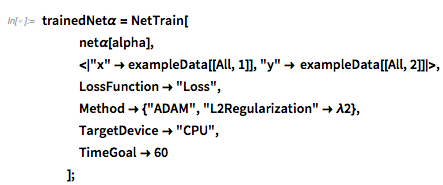✕ ```trainedNet\[Alpha] = NetTrain[ net\[Alpha][alpha], <|"x" -> exampleData[[All, 1]], "y" -> exampleData[[All, 2]]|>, LossFunction -> "Loss", Method -> {"ADAM", "L2Regularization" -> \[Lambda]2}, TargetDevice -> "CPU", TimeGoal -> 60 ];```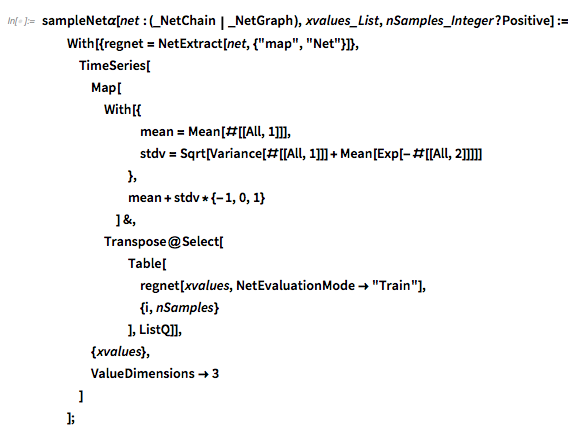✕ ```sampleNet\[Alpha][net : (_NetChain | _NetGraph), xvalues_List, nSamples_Integer?Positive] := With[{regnet = NetExtract[net, {"map", "Net"}]}, TimeSeries[ Map[ With[{ mean = Mean[#[[All, 1]]], stdv = Sqrt[Variance[#[[All, 1]]] + Mean[Exp[-#[[All, 2]]]]] }, mean + stdv*{-1, 0, 1} ] &, Transpose@Select[ Table[ regnet[xvalues, NetEvaluationMode -> "Train"], {i, nSamples} ], ListQ]], {xvalues}, ValueDimensions -> 3 ] ];```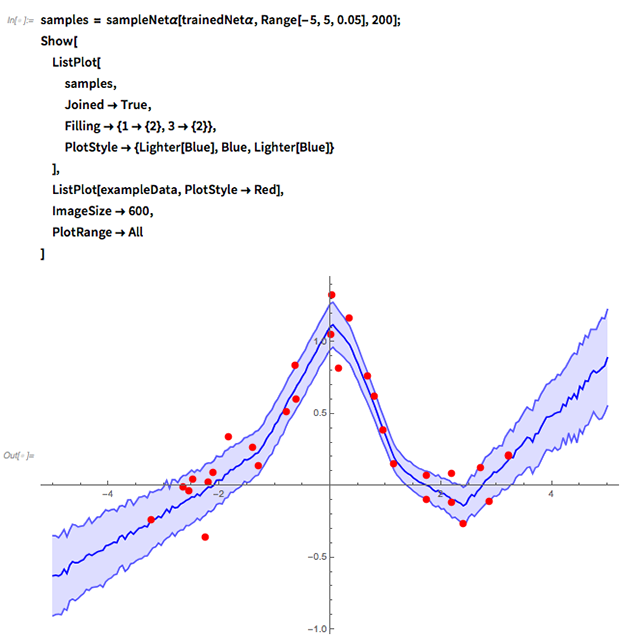✕ ```samples = sampleNet\[Alpha][trainedNet\[Alpha], Range[-5, 5, 0.05], 200]; Show[ ListPlot[ samples, Joined -> True, Filling -> {1 -> {2}, 3 -> {2}}, PlotStyle -> {Lighter[Blue], Blue, Lighter[Blue]} ], ListPlot[exampleData, PlotStyle -> Red], ImageSize -> 600, PlotRange -> All ]```

I’ve discussed that dropout layers and theregularization coefficient in neural network training can actually be seen as components of a Bayesian inference procedure that approximates Gaussian process regression. By simply training a network with dropout layers like normal and then running the network several times in NetEvaluationMode → "Train", you can get an estimate of the predictive posterior distribution, which not only includes the noise inherently in the data but also the uncertainty in the trained network weights.